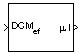Documentation

# Direction Cosine Matrix ECEF to NED to Latitude and Longitude

Convert direction cosine matrix to geodetic latitude and longitude

## Library

Utilities/Axes Transformations## Description

The Direction Cosine Matrix ECEF to NED to Latitude and Longitude block converts a 3-by-3 direction cosine matrix (DCM) into geodetic latitude and longitude. The DCM matrix performs the coordinate transformation of a vector in Earth-centered Earth-fixed (ECEF) axes (ox0, oy0, oz0) into a vector in north-east-down (NED) axes (ox2, oy2, oz2). The order of the axis rotations required to bring this about is:

1. A rotation about oz0 through the longitude (ι) to axes (ox1, oy1, oz1)

2. A rotation about oy1 through the geodetic latitude (μ) to axes (ox2, oy2, oz2)

`$\begin{array}{l}\left[\begin{array}{c}o{x}_{2}\\ o{y}_{2}\\ o{z}_{2}\end{array}\right]=DC{M}_{ef}\left[\begin{array}{c}o{x}_{0}\\ o{y}_{0}\\ o{z}_{0}\end{array}\right]\\ \\ \left[\begin{array}{c}o{x}_{2}\\ o{y}_{2}\\ o{z}_{2}\end{array}\right]=\left[\begin{array}{ccc}-\mathrm{sin}\mu & 0& \mathrm{cos}\mu \\ 0& 1& 0\\ -\mathrm{cos}\mu & 0& -\mathrm{sin}\mu \end{array}\right]\left[\begin{array}{ccc}\mathrm{cos}\iota & \mathrm{sin}\iota & 0\\ -\mathrm{sin}\iota & \mathrm{cos}\iota & 0\\ 0& 0& 1\end{array}\right]\left[\begin{array}{c}o{x}_{0}\\ o{y}_{0}\\ o{z}_{0}\end{array}\right]\end{array}$`

Combining the two axis transformation matrices defines the following DCM.

`$DC{M}_{ef}=\left[\begin{array}{ccc}-\mathrm{sin}\mu \mathrm{cos}\iota & -\mathrm{sin}\mu \mathrm{sin}\iota & \mathrm{cos}\mu \\ -\mathrm{sin}\iota & \mathrm{cos}\iota & 0\\ -\mathrm{cos}\mu \mathrm{cos}\iota & -\mathrm{cos}\mu \mathrm{sin}\iota & -\mathrm{sin}\mu \end{array}\right]$`

To determine geodetic latitude and longitude from the DCM, the following equations are used:

`$\begin{array}{l}\mu =\text{asin}\left(-DCM\left(3,3\right)\right)\\ \\ \iota =\text{atan}\left(\frac{-DCM\left(2,1\right)}{DCM\left(2,2\right)}\right)\end{array}$`

## Parameters

Action for invalid DCM

Block behavior when direction cosine matrix is invalid (not orthogonal).

• Warning — Displays warning and indicates that the direction cosine matrix is invalid.

• Error — Displays error and indicates that the direction cosine matrix is invalid.

• None — Does not display warning or error (default).

Tolerance for DCM validation

Tolerance of direction cosine matrix validity, specified as a scalar. Default is `eps(2)`. The block considers the direction cosine matrix valid if these conditions are true:

• The transpose of the direction cosine matrix times itself equals `1` within the specified tolerance (`transpose(n)*n == 1±tolerance`)

• The determinant of the direction cosine matrix equals `1` within the specified tolerance (`det(n) == 1±tolerance`).

## Inputs and Outputs

InputDimension TypeDescription

First

3-by-3 direction cosine matrixTransforms ECEF vectors to NED vectors.
OutputDimension TypeDescription

First

2-by-1 vectorContains the geodetic latitude and longitude, in degrees.

## Assumptions and Limitations

This implementation generates a geodetic latitude that lies between ±90 degrees, and longitude that lies between ±180 degrees.

The implementation of the ECEF coordinate system assumes that the origin is at the center of the planet, the x-axis intersects the Greenwich meridian and the equator, the z-axis is the mean spin axis of the planet, positive to the north, and the y-axis completes the right-hand system.

## References

Stevens, B. L., and F. L. Lewis, Aircraft Control and Simulation, John Wiley & Sons, New York, 1992.

Zipfel, P. H., Modeling and Simulation of Aerospace Vehicle Dynamics, AIAA Education Series, Reston, Virginia, 2000.

“Atmospheric and Space Flight Vehicle Coordinate Systems,” ANSI/AIAA R-004-1992.

#### Introduced before R2006a

##### SupportGet trial now